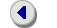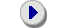### WELCOME TO DUMKALAND

Explicit numerical methods for stiff differential equationsDUMKA3 - integrates initial value problems for systems of first order ordinary differential equations y'=f(y,t). It is based on a family of explicit Runge-Kutta-Chebyshev formulas of order three. It uses optimal third order accuracy stability polynomials with the largest stability region along the negative real axis. The algorithm of construction of high order explicit methods for stiff differential equations described in the article
Alexei A. Medovikov "High order explicit methods for parabolic equations" BIT1998, Vol. 38, No.2, pp.372-390.
In this article the following idea has been formulated: how to "finish sequence of steps to get method of the desired order": if you define a Runge-Kutta method A, then one can define Runge-Kutta method B, so that composition of two methods C=B(A) (method B used after method A) is the method order p.
For example, described in this article is the algorithm of construction of s-stage (number of RHS evaluations) and p-order explicit method is based on s-p Euler steps (method A) and explicit p-stage remainder B, so that composition of these two methods (method C=B(A)) becomes a method of order p. To choose explicit Euler steps (method A) I take a p-order optimal stability polynomial degree s, then I take out p roots from this polynomial and the rest of roots made up sequence of Euler steps for method A, the reminder method (B) finish construction of optimal stability polynomial, so that method C has optimal stability polynomial I've chosen, and at the same time lead to p-order composition method C=B(A). As the result I have p order method (for non-liner problems) with optimal stability polynomial. The same idea was used for construction fourth order explicit solvers dumka4 and rock4.

These formulas have been derived based on theory of composition methods. To read about composition methods I would recommend the book of
Ernst Hairer Syvert Paul Nørsett Gerhard Wanner Solving Ordinary Differential Equations I. Nonstiff Problems. Springer Series in Comput. Mathematics, Vol. 8, Springer-Verlag 1987, Second revised edition 1993.
and
Ernst Hairer Gerhard Wanner Solving Ordinary Differential Equations II. Stiff and Differential-Algebraic Problems. Springer Series in Comput. Mathematics, Vol. 14, Springer-Verlag 1991, Second revised edition 1996.
Professors Ernst Hairer and Gerhard Wanner have contributed several ideas into dumka3, especially I would recommend reading about embedded formulas, this algorithm allow efficient step-size control with just a minor increasing of computational efforts.

Perhaps the major reason stable explicit methods have not been used for so long is the problem with internal stability of these methods, which is closely related to the similar problem for Chebyshev iterative methods: inspite of the fact that Chebyshev polynomial is stable, computation of high degree Chebyshev polynomial may be unstable, because intermediate polynomials can be large. This problem was overcome by an algorithm proposed by Lebedev and Finogenov for Chebyshev iterative methods, which is based on special sequence of roods of Chebyshev polynomials. This "special sequence" forces  intermediate polynomials to be shifted Chebyshev polynomials, so that intermediate results are obtained from stable polynomials as well. Ordering of parameters in DUMKA3 was done mostly by V.I. Lebedev and of course it would be interesting to read articles of my scientific advisor - Professor V.I. Lebedev.

The problem of internal instability can be overcome by using of recurrent relations for orthogonal polynomials. Initially this idea was formulated for Chebyshev iterative methods, then it was used for explicit ODE solvers. For more details please refer to articles of Professors van der Houwen, B.P. Sommeijer, L.F. Shampaine, J.G. Verwer.

Because of this wonderful idea and because of works of professor Lebedev on orthogonal polynomials which approximate minimal polynomials with weight functions, we come up with idea of using recurrent relations for orthogonal polynomials different from Chebyshev polynomials, which approximate polynomials of least deviation from zero with a weight. If we choose the weight to be also a polynomial then the product of the weight and the polynomial is a polynomial, but this product of the polynomial and weight function is close to a polynomial of least deviation from zero which approximates the exponential function in the vicinity of the origin used by dumka2, dumka3 and dumka4. So, we have calculated weights for different degrees of almost optimal (but orthogonal) stability polynomials and use reccurent relation to build final polynomial and then multiply by weight. All intermediate stages of this reccurent process are stable and as the result we do not have intermediate instability. For details I would highly recommend to read the article
Assyr Abdulle, Alexei A. Medovikov: Second order Chebyshev methods based on orthogonal polynomials Numer. Math. 90 (2001) 1, 1-18, and PhD of Assyr Abdulle, where he developed these ideas for fourth order explicit methods based on orthogonal polynomials ( Abdulle A: Fourth order Chebyshev methods with recurrence relation SIAM J SCI COMPUT 23 (6): 2041-2054 MAY 17 2002).